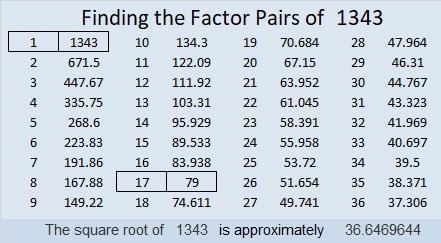# 1343 Level TWO Puzzles OnlyLevel 2 puzzles are much more interesting-looking than level 1 puzzles, but they are still relatively easy for beginners to solve. I decided to put all the level 2 puzzles from 2018 into one collection. You can use the image I put at the top of the post to work on solving them or you can find the complete collection at Level 2’s from 2018.

Now I’ll write a little bit about the number 1343:

• 1343 is a composite number.
• Prime factorization: 1343 = 17 × 79
• 1343 has no exponents greater than 1 in its prime factorization, so √1343 cannot be simplified.
• The exponents in the prime factorization are 1, and 1. Adding one to each exponent and multiplying we get (1 + 1)(1 + 1) = 2 × 2 = 4. Therefore 1343 has exactly 4 factors.
• The factors of 1343 are outlined with their factor pairs in the graphic below.1343 is the hypotenuse of a Pythagorean triple:
632-1185-1343 which is (8-15-17) times 79

Stetson.edu informs us that 1343 is 16 numbers away from the closest prime number, and it is the smallest number that can make that claim.